# Langly Extrapolation¶

## My Summer on the volcano¶

I'm going to write about my 2018 Summer. This post is all about my time at NOAA's Mauna Loa Station. I began measuring ozone for the world's standard Dobson Spectrophotometer (083) in June 2018 and stayed until October 31, 2018. I measured through the Kilauea volcanic eruption and was there when we were hit by Hurricane Lane. It was quite an honor performing this calibration and was an experience working at 11000 feet above sea level. Here are some pictures of where I worked

Here is me climbing a 30-meter tower. I'm actually clinging for dear life. The wind would sway the tower. The NOAA staff I was helping remove equipment on the tower probably wouldn't have let me up there if they knew how scared I really was.Here is a view of the station from a 30 meter tower.Here is a photo of me posing with the Dobson Spectrophotometer.Most of my time involved gathering data. I would wake up at 4:30 in the morning and hit the road by five, driving through the Big Island's fields and forest. I started at about 700' and climbed up to 11,141'. Each day took about 2.5 hours of driving.

Once up there I would set up the Dobson and run observations from about 6:45 to 10:30. Afterward, I would roll the Dobson back inside and perform some routine tests. The day would end with me transmitting the data to the NOAA Global Monitoring Division Headquarters in Boulder Colorado. From there I would drive back for a well-earned nap.

Four months of repetitive measurement are over. The whole point of my internship was to gather data needed for Langley extrapolation. It is a method used to estimate the intensity of sunlight at the top of the Earth before it reaches the ozone layer. This intensity is used as a calibration constant used by many other Dobson Spectrophotometers utilized worldwide.

The remaining section of this post shows us how to extrapolate this value, known as the extraterrestrial solar constant using Python.

## Theory¶

Let us define the ground based intensity of light as $I$. Then the logarithmic ratio of intensity between two wavelength pairs are devined as $$L_{\mu} = log \left( \frac{I_{\mu}}{I^{'}_{\mu}} \right)$$

Where $\mu$ is a ratio of actual and vertical paths of solar radiation. You may think of it as a measurement of how much atmosphere sunlight travels through. Here we denote $I_{\mu}$ and $I^{'}_{\mu}$ as short and long wavelength intensity respectively.

As the morning progresses, $\mu$ gets smaller with time, but never less than 1. $\mu=1$ is one atmosphere worth of air. Before increasing as the afternoon progresses.

Suppose we are interested in measuring the intensity of light before it travels through the atmosphere, $L_{0}$? For this scenario $\mu=0$, which is impossible to measure on the Earth's surface directly. Instead, we rely on a particular type of extrapolation called Langley extrapolation, which creates a curve of some fitted function vs. $\mu$ and extrapolates that function to $\mu=0$. The fitted function we have in mind is called $P^{*}$.

Given $I_{\mu}$, we can plot out the day's sunlight intensity. Modeling this process as a function of $\mu$, along with some transformations, this introduced parameter $P^{*}$ is a straight line with respect to $\mu$. From there we extrapolate the curve formed by observational data $P^{*}(\mu)$ when $\mu=0$. The theoretical curve for $P^{*}(\mu)$ is

$$P^{*}(\mu) = \frac{L^{*}_{0} - L_{\mu} - (\beta - \beta')m}{\mu}$$

Where $L^{*}_{0}$ is a rough idea of the extraterrestrial constant. $\beta$ and $\beta'$ are the Rayleigh scattering coefficients of air at the short and long wavelengths, respectively; and m is the ratio of the actual and vertical paths of solar radiation through the atmosphere, taking into account refraction and the earth's curvature: airmass;

According to Dobson, $P^{*}(\mu)$ should be a horizontal straight line. If not, then a curved line will result. Modifying $L^{*}_{0}$ can be adjusted until a straight line is created, providing us with the new estimate for $L^{*}_{0}$.

This derivation is described further in Dobson and Normand's 1958 paper: "Determination of Constants etc. Used in the Calculation of the Amount of Ozone from Spectrophotometer Measurements and an Analysis of the Accuracy of the Results."

Going forward, know that we have a recursive method to find the extraterrestrial constant $L^{*}_{0}$

### Parameters¶

Komhyr offers us several useful parameters used by the expression for $P^{*}(\mu)$ in his 1980 handbook "Ozone Operations with a Dobson Spectrophotometer." Page 34 gives an expression for m, taken from Hither & Hardie, 1962.

$$m = sec(SZA) - 0.0018167 (sec(SZA) - 1) - 0.002875 (sec(SZA) - 1)^2 - 0.0008083 * (sec(SZA) -1 )^3$$

Where SZA is the solar zenith angle.

Table 5 on page 32 gives values for $(\beta - \beta')$ for several wavelengths and wavelength pairs. Below is a truncated table showing values for A, B, C, and D wavelength pairs.

Wavelength Pair Rayleigh scattering coefficients $\beta-\beta'$
A .114
B .111
C .109
D .104

We don't have to know what $\mu$ is. The program records it for us; but, I provided it here from page 32.

The quantity $\mu$ represents the ratio of the actual path length of a ray of light through the Ozone layer as compared to the vertical path length. It is computed from the equation:

$$\mu = \frac{R + h}{\sqrt{( R + h )^2 − ( R + r )^2 sin^2 ( SZA )}}$$

where R = mean earth radius (6371.229 km); r = height of the station above mean sea level; h = height of the ozone layer above mean sea level at the location of the station

## Data¶

The data output that Dobson Spectrophotometer client produces will is a little different. For instance, instead of giving us $L$, it outputs $N = L^{*}_{0} - L$ going forward, we will use the expression.

$$P^{*}(\mu) = \frac{N_{\mu} - (\beta - \beta')m}{\mu}$$

Here we have C, D, and A wavelength observations, made by taking measurements from $\mu=5$ till approximately $\mu=1.15$. Measurements are recorded for a particular time, and from that time, $\mu$ is calculated. $N_{\mu}$ is also calculated by converting spectrophotometer encoder counts into $N$ using a wedge calibration table. Here, an estimated $L_0$ is used; most likely, the determined value the last time such a measurement was made.

We shall now view a typical measurement for P

In :
import os
import pandas as pd
import glob
import numpy as np
import pdb
from scipy import stats
import seaborn as sns
import matplotlib
from matplotlib import rc
from matplotlib import rcParams
import matplotlib.ticker as mtick
import matplotlib.pyplot as plt
rc('text', usetex=False)
rcStyle = {"font.size": 10,
"axes.titlesize": 14,
"axes.labelsize": 14,
'xtick.labelsize': 10,
'ytick.labelsize': 10}
sns.set_context("paper", rc=rcStyle)
sns.set_style("whitegrid", {'axes.grid' : False})
myColors = ["windows blue", "dusty rose", "amber", "greyish", "faded green", "dusty purple"]
sns.set_palette(sns.xkcd_palette(myColors))
%matplotlib inline
HOMEDIR = os.getcwd()
files = []
files = files + glob.glob(os.path.join(DATADIR, 'CDAobs*'))

file = files
df.columns = [ col.replace(' ', '') for col in df.columns.tolist() ]
date = df.DateUTC
print(date)

2018-09-27


From here we can create plots of $P^*(\mu)$

In :
sec = lambda sza: 1/np.cos(sza)
get_m = lambda sza: sec(sza) - 0.0018167*(sec(sza) - 1) - 0.002875*(sec(sza) - 1)**2 - 0.0008083 * (sec(sza) -1 )**3

def get_betaMbeta(wavePair):
if wavePair == 'A':
return .114
elif wavePair == 'B':
return .111
elif wavePair == 'C':
return .109
elif wavePair == 'D':
return .104

def create_plot_of_P(dfIn, wavePair, outliers=[]):
df = dfIn
for idx in outliers:
df = df.drop(idx)
nLab = 'N' + wavePair.lower()
szaLab = 'SZA_at_time_for_' + wavePair
xLab = 'Mu_at_time_for_' + wavePair
pLab = 'P' + wavePair.lower()
N = df[nLab].astype(float)
betambetam = get_betaMbeta(wavePair) * df[szaLab].astype(float).apply(get_m)
df[pLab] = (N - betambetam) / df[xLab].astype(float)
fig = plt.figure()
axes = plt.axes()
axes.plot(df[pLab].values, linewidth = 2)
#axes.plot(df[xLab].values,df[pLab].values, linewidth = 2)
axes.set_title('$P^{*}$ for wavelength pair: '+wavePair+' vs. $\mu$')
axes.set_ylabel('$P^{*}$')
axes.set_xlabel('index')

In :
create_plot_of_P(df, 'A')
create_plot_of_P(df, 'C')
create_plot_of_P(df, 'D')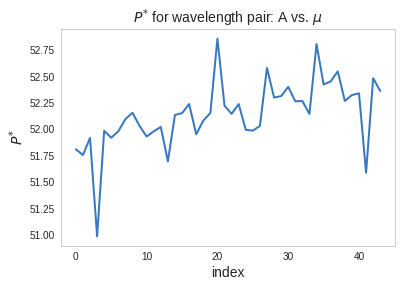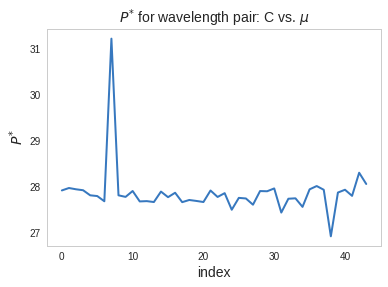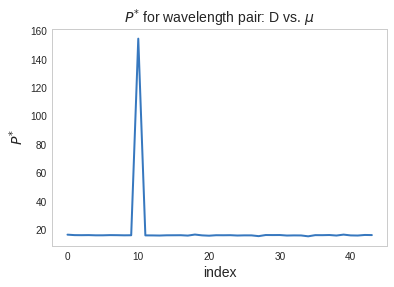Dobson Spectrophotometer Measurement errors are easy to spot, as shown for wavelength Pair D. the 10th point (starting from zero) shall be removed.

## Determining correction factor $\phi$¶

According to Dobson, plotting $P^{*}(\mu)$ vs. $\mu$ should yield a horizontal straight line if $L_o$ is accurate. If not then a correction factor $\phi$ can be used to flatten out the line.

$$P^{*}(\mu, \phi) = \frac{N_{\mu} + \phi - (\beta - \beta')m}{\mu}$$

Back when Dobson and Normand's paper was written, the technique used involved drawing lines and graphing. Today we can perform a simple linear regression on $P^{*}(\mu, \phi)$, and find the value $\phi$ such that the slope of the fitted curve is zero.

Here we are fitting $P^{*}(\mu, \phi)$ as a line with slope $m$ and intercept $b$

$$\hat{P^{*}}(\mu, \phi) = m \mu + b$$

We don't need to worry about b. Digging through a stats book, I found

$$m = \frac{Cov(P^{*},\mu)}{var(\mu)}$$

Where $Cov(P^{*},\mu) = \Sigma^n_{i=1} P^{*}\mu - \Sigma^n_{i=1} P^{*}\Sigma^n_{i=1} \mu$.

For a flat slope such that $m$ = 0, occuring when $Cov(P^{*},\mu)=0$.

$$Cov(P^{*},\mu) = \Sigma^n_{i=1} P^{*}_i\mu - \Sigma^n_{i=1} P^{*}_i\Sigma^n_{i=1} \mu = 0$$

$$\Sigma^n_{i=1} P^{*}_i\mu_i = \Sigma^n_{i=1} P^{*}_i\Sigma^n_{i=1} \mu_i$$

$$\Sigma^n_{i=1} \left( N_i + \phi - (\beta - \beta')m_i \right) = \Sigma^n_{i=1} \mu_i \Sigma^n_{i=1} \left( \frac{N_i + \phi - (\beta - \beta')m_i}{\mu_i} \right)$$

Distributing the summation and solving for $\phi$ gives us

$$\phi = \frac{ \bar{\mu}\left(\Sigma^n_{i=1} \frac{N_i - (\beta - \beta')m_i }{\mu_i} \right) - \Sigma^n_{i=1} N_i + \Sigma^n_{i=1}(\beta - \beta')m_i }{(n -\bar{\mu}\Sigma^n_{i=1} \frac{1}{\mu_i})}$$

where $\bar{\mu} = \frac{\Sigma^n_{i=1}\mu_i}{n}$

In :
def get_optimized_phi(dfIn, wavePair, outliers=[]):
df = dfIn
for idx in outliers:
df = df.drop(idx)

nLab = 'N' + wavePair.lower()
szaLab = 'SZA_at_time_for_' + wavePair
pLab = 'P' + wavePair.lower()
xLab = 'Mu_at_time_for_' + wavePair
N = df[nLab].astype(float)
numSample = N.shape
mu  = df[xLab].astype(float)
gamma = get_betaMbeta(wavePair) * df[szaLab].astype(float).apply(get_m)

P = (N - gamma) / mu
numer = mu.mean() * ( P.sum() ) - N.sum() + gamma.sum()
#denom =  numSample * ( 1 - mu.mean() * mu.apply(lambda x: 1/x).sum() )
denom =  ( numSample - mu.mean() * mu.apply(lambda x: 1/x).sum() )
phi = numer / denom
return phi

In :
PhiOptA = get_optimized_phi(df, 'A')
PhiOptC = get_optimized_phi(df, 'C')
PhiOptD = get_optimized_phi(df, 'D', outliers=)

print(PhiOptA)
print(PhiOptC)
print(PhiOptD)

-1.2150943796249616
0.6591078458414585
0.3264047352106591

In :
def get_P(df, phi, wavePair):
nLab = 'N' + wavePair.lower()
szaLab = 'SZA_at_time_for_' + wavePair
pLab = 'P' + wavePair.lower()
xLab = 'Mu_at_time_for_' + wavePair
N = df[nLab].astype(float)
numSample = N.shape
mu  = df[xLab].astype(float)
gamma = get_betaMbeta(wavePair) * df[szaLab].astype(float).apply(get_m)
P = (N + phi - gamma) / mu
return P

def create_plot_of_P_with_phi(dfIn, phi, wavePair, outliers=[]):

df = dfIn
for idx in outliers:
df = df.drop(idx)
xLab = 'Mu_at_time_for_' + wavePair
pLab = 'P_for_' + wavePair
pFit = 'P_fit_for_' + wavePair
df[pLab] = get_P(df, phi, wavePair)
df[xLab] = df[xLab].astype(float)
linreg = stats.linregress(df[xLab],df[pLab])
df[pFit] = df[xLab].apply(lambda x: x * linreg.slope + linreg.intercept)
fig = plt.figure()
axes = plt.axes()
axes.plot(df[xLab].values,df[pLab].values, linewidth = 2)
axes.plot(df[xLab].values, df[pFit].values, linewidth = 2)
axes.set_title('$P^{*}$ for wave pair '+wavePair+' vs. $\mu$')
axes.set_ylabel('$P^{*}$')
axes.set_xlabel('$\mu$')
axes.set_xlim([df[xLab].values.min(),df[xLab].values.max()])
axes.legend(['Data', r'Fit'], frameon = True)

create_plot_of_P_with_phi(df, PhiOptA,  'A')
create_plot_of_P_with_phi(df, PhiOptC,  'C')
create_plot_of_P_with_phi(df, PhiOptD,  'D', outliers=)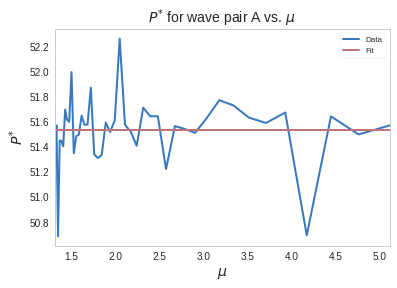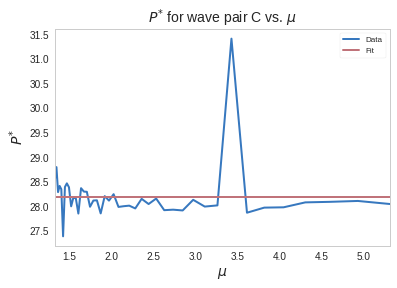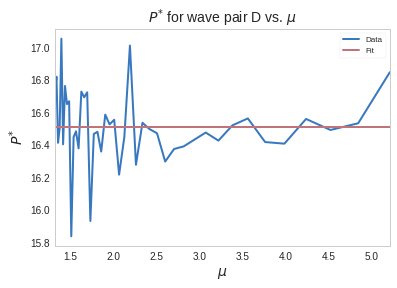## Correction factor time series.¶

I repeated this process for each day I measured Langley plots, creating this timeseries.## Discussion¶

I'm suspicious of the following dates:

date reason
2018-10-11 Had to move Dobson due to building blocking the sun
2018-07-03 plot curves up
2018-07-05 progressivly more cloudy
2018-09-19 partial day, too cloudy
2018-07-12 partial day, too cloudy
2018-08-06 wildfire smoke drifted from Waikoloa. computer also froze on this day

The correction factor $\phi$ varies by alot, but is generally safe.

There is a noticble dip around August 30th. We adjusted the Q-table around this time, which could explain why the correction factor dips slightly. Q-tables are used to account for temperature variation and are calibrated every few years. The Dobson I was using was updated at this time. We should expect to see different measurements as a result. This is however correctable and I'm working on finding out how.

## Conclusion¶

This post is still a work in progress. I haven't written this yet